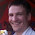## Friday, October 21, 2011

### Project Euler Problem 2 in Scala

This is 2 in a series.  The Previous post is at, Project Euler and Scala Problem 1.

Here is the second problem on the Project Euler(PE) site.

Each new term in the Fibonacci sequence is generated by adding the previous two terms. By starting with 1 and 2, the first 10 terms will be:
1, 2, 3, 5, 8, 13, 21, 34, 55, 89, ...
By considering the terms in the Fibonacci sequence whose values do not exceed four million, find the sum of the even-valued terms.

Once again, I was able to solve this pretty easily, but I had lots of mutable state in my code.  Here was my first solution:

object Problem2 {
val fourMillion : Integer = 4000000;

var first : Integer = 0;
var second : Integer = 1;

def nextFib() : Integer = {
var returnValue:Integer = prev1+prev2;
return returnValue;
}

var sum : Long = 0;

def main(args: Array[String]): Unit = {
var next:Integer = 0;
while( (next <= fourMillion) ) {
next = nextFib();
if(next % 2 == 0) {
sum = sum + next;
}

}
print(sum)
}
}

You will see that I have 3 mutable variables in there -  first, second, and sum.  I also have a rather ugly while loop.  I'd like to get rid of the iteration here and use recursion instead.  While doing so, I can also get rid of the mutable state.  Here is the rewritten code:

object Problem2 {
//Tail recursive...
def fibList(currentList : Array[Int], limit : Int, previous : Int) : Array[Int] = {
if(previous > limit) {
return currentList;

return fibList((currentList :+ previous), limit, (currentList.last + previous));
}

def main(args: Array[String]): Unit = {
print((0 /: fibList(Array[Int](0,1), 4000000, 1))((total, next)=> {
if(next % 2 == 0) {
next + total
} else {
total
}
}))
}
}

I hope you notice the comment in there - Tail Recursive.  This is a very interesting feature of Scala, and most other functional languages.  You see, the big concern in Java when using recursion is that you run out of stack space for all your recursions.  In Scala, the compiler can optimize this code and get rid of the stack if you don't need to keep state in your method.  I've carefully constructed the fibList here so that it can be optimized.  This way, we can't fill up the stack.  go ahead, change 4000000 to 4000000000.  While you may overflow the int values, the recursive function won't blow up the memory.

This feature of the language is really quite interesting, and from my understanding not a small feat to pull off given the current bytecode standard.  This could allow for some very long-running calculations that might otherwise have caused memory problems if done other ways.

That's all for this problem.  On to #3...

1.this is my version using list, because list are fun

object Euler2{

def fibo(pList : List[Long]) : List[Long] = {
pList match {
case Nil => List(1)
case 1::Nil => List(2,1)
case a::b => pList.take(2).sum :: pList
}
}

def main(args : Array[String]) : Unit = {

var list : List[Long]= List(1)
val limit = 4 * 1000 * 1000

list = fibo(list)
}

list = list.tail
val sum = list.filter(x => x%2 == 0).sum

print("sum is " + sum)

}

}

2.HI renghen. I particularly like your use of the API methods - head, sum, match, filter. I hope to become more familiar with the API as I work through the solutions.